Study Materials: ncert solutions

Our ncert solutions for Exercise 1.3 Class 10 maths 1. Real Numbers - ncert solutions - Toppers Study is the best material for English Medium students cbse board and other state boards students.

Solutions ⇒ Class 10th ⇒ Mathematics ⇒ 1. Real Numbers

# Exercise 1.3 Class 10 maths 1. Real Numbers - ncert solutions - Toppers Study

Topper Study classes prepares ncert solutions on practical base problems and comes out with the best result that helps the students and teachers as well as tutors and so many ecademic coaching classes that they need in practical life. Our ncert solutions for Exercise 1.3 Class 10 maths 1. Real Numbers - ncert solutions - Toppers Study is the best material for English Medium students cbse board and other state boards students.

## Exercise 1.3 Class 10 maths 1. Real Numbers - ncert solutions - Toppers Study

CBSE board students who preparing for class 10 ncert solutions maths and Mathematics solved exercise chapter 1. Real Numbers available and this helps in upcoming exams 2023-2024.

### You can Find Mathematics solution Class 10 Chapter 1. Real Numbers

• All Chapter review quick revision notes for chapter 1. Real Numbers Class 10
• NCERT Solutions And Textual questions Answers Class 10 Mathematics
• Extra NCERT Book questions Answers Class 10 Mathematics
• Importatnt key points with additional Assignment and questions bank solved.

NCERT Solutions do not only help you to cover your syllabus but also will give to textual support in exams 2023-2024 to complete exercise 1.3 maths class 10 chapter 1 in english medium. So revise and practice these all cbse study materials like class 10 maths chapter 1.3 in english ncert book. Also ensure to repractice all syllabus within time or before board exams for ncert class 10 maths ex 1.3 in english.

See all solutions for class 10 maths chapter 1 exercise 1 in english medium solved questions with answers.

### Exercise 1.3 class 10 Mathematics Chapter 1. Real Numbers

• Exercise 1.3 Class 10 Maths 1. Real Numbers - Ncert Solutions - Toppers Study
• Class 10 Ncert Solutions
• Solution Chapter 1. Real Numbers Class 10
• Solutions Class 10
• Chapter 1. Real Numbers Exercise 1.3 Class 10

## Exercise 1.3 Class 10 maths 1. Real Numbers - ncert solutions - Toppers Study

Exercise 1.3

Q1. Prove that √5 is irrational.

Q2. Prove that 3 + 2√5 is irrational.

Q3. Prove that the following are irrationals:

 (i) 1 √2

(ii)7√ 5

(iii)6 +√ 2

NCERT Exercise Solutions:

### Exercise 1.3 Class 10 maths Chapter 1. Real Numbers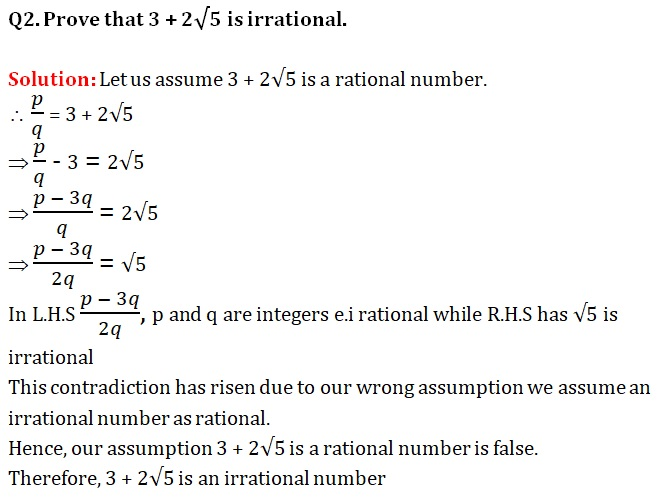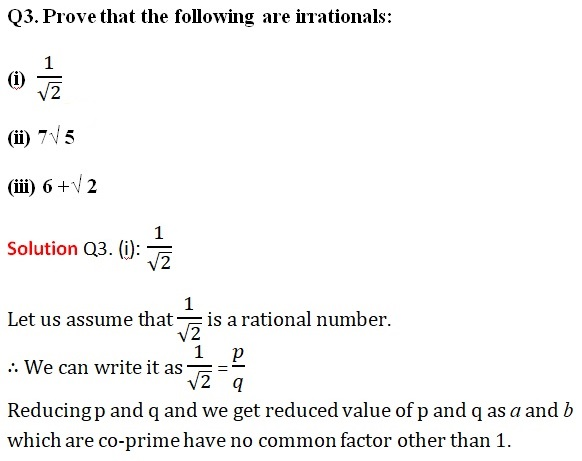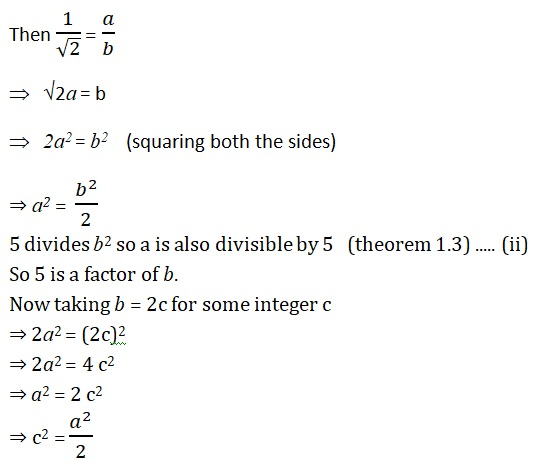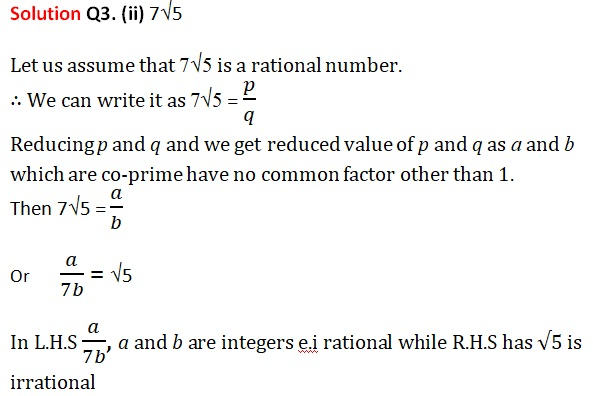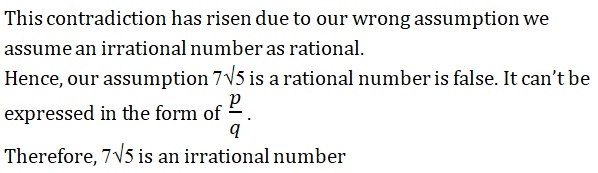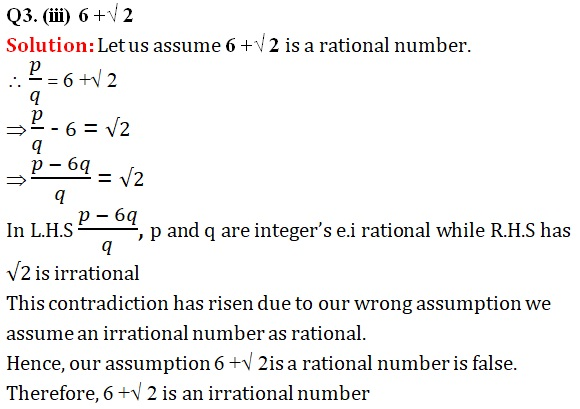##### Other Pages of this Chapter: 1. Real Numbers

Important Study materials for classes 06, 07, 08,09,10, 11 and 12. Like CBSE Notes, Notes for Science, Notes for maths, Notes for Social Science, Notes for Accountancy, Notes for Economics, Notes for political Science, Noes for History, Notes For Bussiness Study, Physical Educations, Sample Papers, Test Papers, Mock Test Papers, Support Materials and Books.Mathematics Class - 11th

NCERT Maths book for CBSE Students.

books

## Study Materials List:

##### Solutions ⇒ Class 10th ⇒ Mathematics
1. Real Numbers
2. Polynomials
3. Pair of Linear Equations in Two Variables
5. Arithmetic Progressions
6. Triangles
7. Coordinate Geometry
8. Introduction to Trigonometry
9. Some Applications of Trigonometry
10. Circles
11. Constructions
12. Areas Related to Circles
13. Surface Areas and Volumes
14. Statistics
15. Probability

New Books